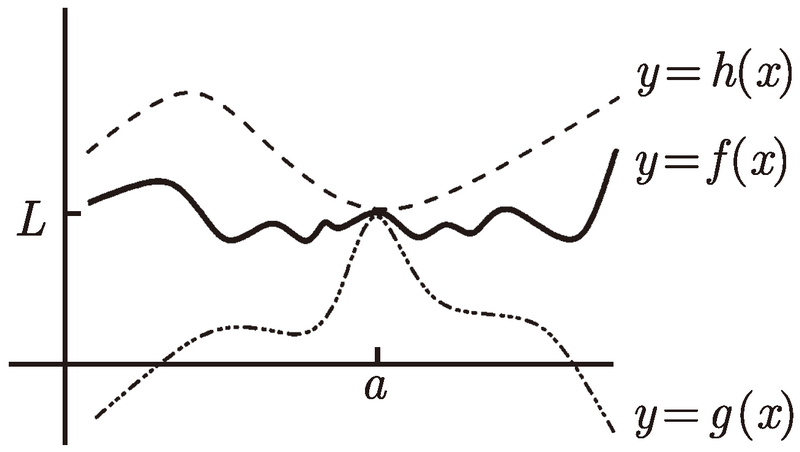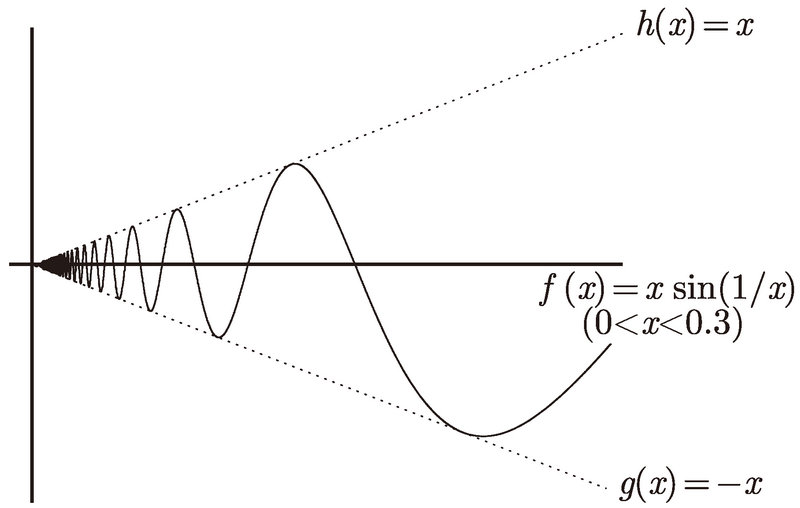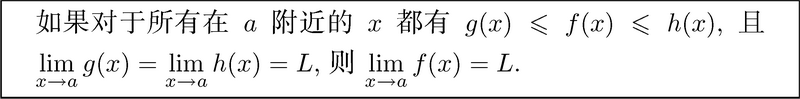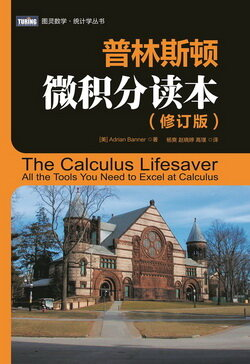# 普林斯顿微积分读本（修订版）(26)：极限导论 3.6(三明治定理)$\lim_{x\to0^+}x\sin\biggl(\frac{1}{x}\biggr)$$-1\leq\sin\biggl(\frac{1}{x}\biggr)\leq1.$

$-x\leq x\sin\biggl(\frac{1}{x}\biggr)\leq x.$

$\lim_{x\to0^+}g(x)=\lim_{x\to0^+}(-x)=0$　及　$\lim_{x\to0^+}h(x)=\lim_{x\to0^+}x=0$.

$\lim_{x\to0^+}x\sin\biggl(\frac{1}{x}\biggr)=0.$

$\lim_{x\to\infty}\frac{\sin(x)}{x}=0.$

$-\frac{1}{x}\leq\frac{\sin(x)}{x}\leq\frac{1}{x}.$

$\lim_{x\to\infty}-\frac{1}{x}=0$　和　$\lim_{x\to\infty}\frac{1}{x}=0$,

$\lim_{x\to\infty}\frac{\sin(x)}{x}=0.$